# Banach indicatrix

multiplicity function, of a continuous function $y=f(x)$, $a\leq x\leq b$

An integer-valued function $N(y,f)$, $-\infty < y < \infty$, equal to the number of roots of the equation $f(x)=y$. If, for a given value of $y$, this equation has an infinite number of roots, then $$N(y,f) = +\infty,$$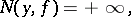and if it has no roots, then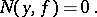The function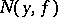was defined by S. Banach  (see also ). He proved that the indicatrixof any continuous function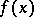in the interval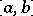is a function of Baire class not higher than 2, and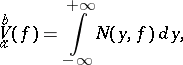(*)

whereis the variation ofon. Thus, equation (*) can be considered as the definition of the variation of a continuous function. The Banach indicatrix is also defined (preserving equation (*)) for functions with discontinuities of the first kind . The concept of a Banach indicatrix was employed to define the variation of functions in several variables , .